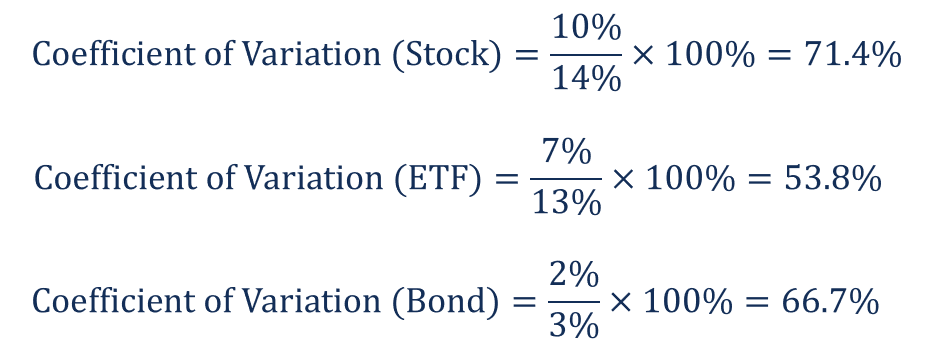# Coefficient of Variation

A statistical measure of the dispersion of data points around the mean

## What is the Coefficient of Variation?

The coefficient of variation (relative standard deviation) is a statistical measure of the dispersion of data points around the mean. The metric is commonly used to compare the data dispersion between distinct series of data. Unlike the standard deviation that must always be considered in the context of the mean of the data, the coefficient of variation provides a relatively simple and quick tool to compare different data series.In finance, the coefficient variation is important in investment selection. From a financial perspective, the financial metric represents the risk-to-reward ratio where the volatility shows the risk of an investment and the mean indicates the reward of an investment.

By determining the coefficient of variation of different securities, an investor identifies the risk-to-reward ratio of each security and develops an investment decision. Generally, an investor seeks a security with a lower coefficient (of variation) because it provides the most optimal risk-to-reward ratio with low volatility but high returns. However, the low coefficient is not favorable when the average (expected return) is below zero.

### Formula for Coefficient of Variation

Mathematically, the standard formula for the coefficient of variation is expressed in the following way:Where:

• σ – the standard deviation
• μ – the mean

In the context of finance, we can re-write the above formula in the following way:### Example of Coefficient of Variation

Fred wants to find a new investment for his portfolio. He is looking for a safe investment that provides stable returns. He considers the following options for investment:

• Stocks: Fred was offered stocks of ABC Corp. It is a mature company with the strong operational and financial performance. The volatility of the stock is 10% and the expected return is 14%.
• ETFs: Another option is the Exchange-Traded Fund (ETF), which tracks the performance of the S&P 500 index. The ETF offers an expected return of 13% with a volatility of 7%.
• Bonds: Bonds with excellent credit ratings offer an expected return of 3% with a 2% volatility.

In order to select the most suitable investment opportunity, Fred decided to calculate the coefficient of variation of each option. Using the formula above, he obtained the following results:Based on the calculations above, Fred wants to invest in ETF because it offers the lowest coefficient (of variation) and the most optimal risk-to-reward ratio.

### Related Readings

CFI offers the Financial Modeling & Valuation Analyst (FMVA)™ certification program for those looking to take their careers to the next level. To keep learning and advancing your career, the following resources will be helpful:

• Investing: A Beginner’s Guide
• Index Funds
• Portfolio Manager
• Systemic Risk

### Financial Analyst Certification

Become a certified Financial Modeling and Valuation Analyst (FMVA)® by completing CFI’s online financial modeling classes and training program!